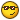# Curious if anyone else knows (Linear Algebra question)

## Recommended Posts

An issue I don't really understand in my linear algebra class is where I'm supposed to show that

A) Show T is a linear transformationFind a basis for the Kernel of T

C) Find a basis for the range of T

Given that

Pn denote polys of degree less than or equal to n, where N > 2.

by T: Pn -> Pn by T(f(x)) = x^2 * F''(x) (second derive)

I understand that the dimension of Pn is = n +1 and when you take the second derivative the dim = n -1 since you are deriving twice from a polynomial. But what about the (x^2) multiplication? does that increase the dim by 2? making it back to the original dim = n +1?

Does that make the basis for the range of T the monomial basis? of (1, x , x^2, x^3.... x^n)?

I'm not entirely sure how to find a basis for the kernel or range. Or prove the linear transformation.

I've been looking around other places and can't really find anything, nor my textbooks provides any useful information.